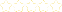• > Ian VanderSchee
• > 5. Derivatives of Trigonometric Functions and Related Rates
+# 5. Derivatives of Trigonometric Functions and Related Rates

Owner: Ian VanderSchee
##### Description:
The derivatives of trigonometric functions are discussed and applied. For HL learners, the derivatives of reciprocal trigonometric and inverse trigonometric functions are also applied, along with problems involving related rates.

### Your Quiz Points in this Playlist

Total Possible
0
5 Tutorials in this Playlist

#### 5.1 Derivatives of Trigonometric Functions

Author: Ian VanderSchee
Rating:(2)
This tutorial has a quiz
•• -

#### 5.2 [HL Only] Derivatives of Reciprocal Trigonometric Functions

Author: Ian VanderSchee
Rating:(0)
This tutorial has a quiz
•• -

#### 5.3 [HL Only] Derivatives of Inverse Trigonometric Functions

Author: Ian VanderSchee
Rating:(1)
This tutorial has a quiz
•• -

#### 5.4 Maxima and Minima with Trigonometry

Author: Ian VanderSchee
Rating:(2)
This tutorial has a quiz
•• -

#### 5.5 [HL Only] Related Rates

Author: Ian VanderSchee
Rating:(0)
This tutorial has a quiz
•• -